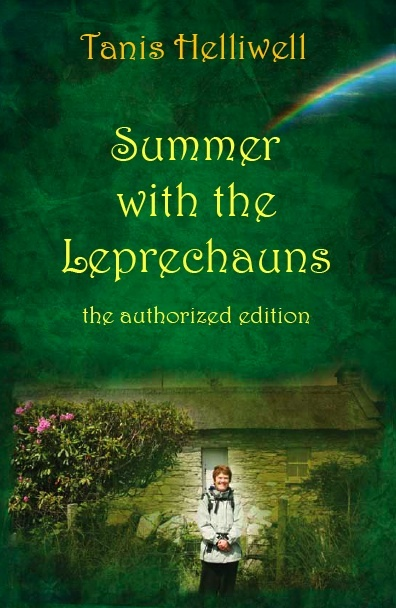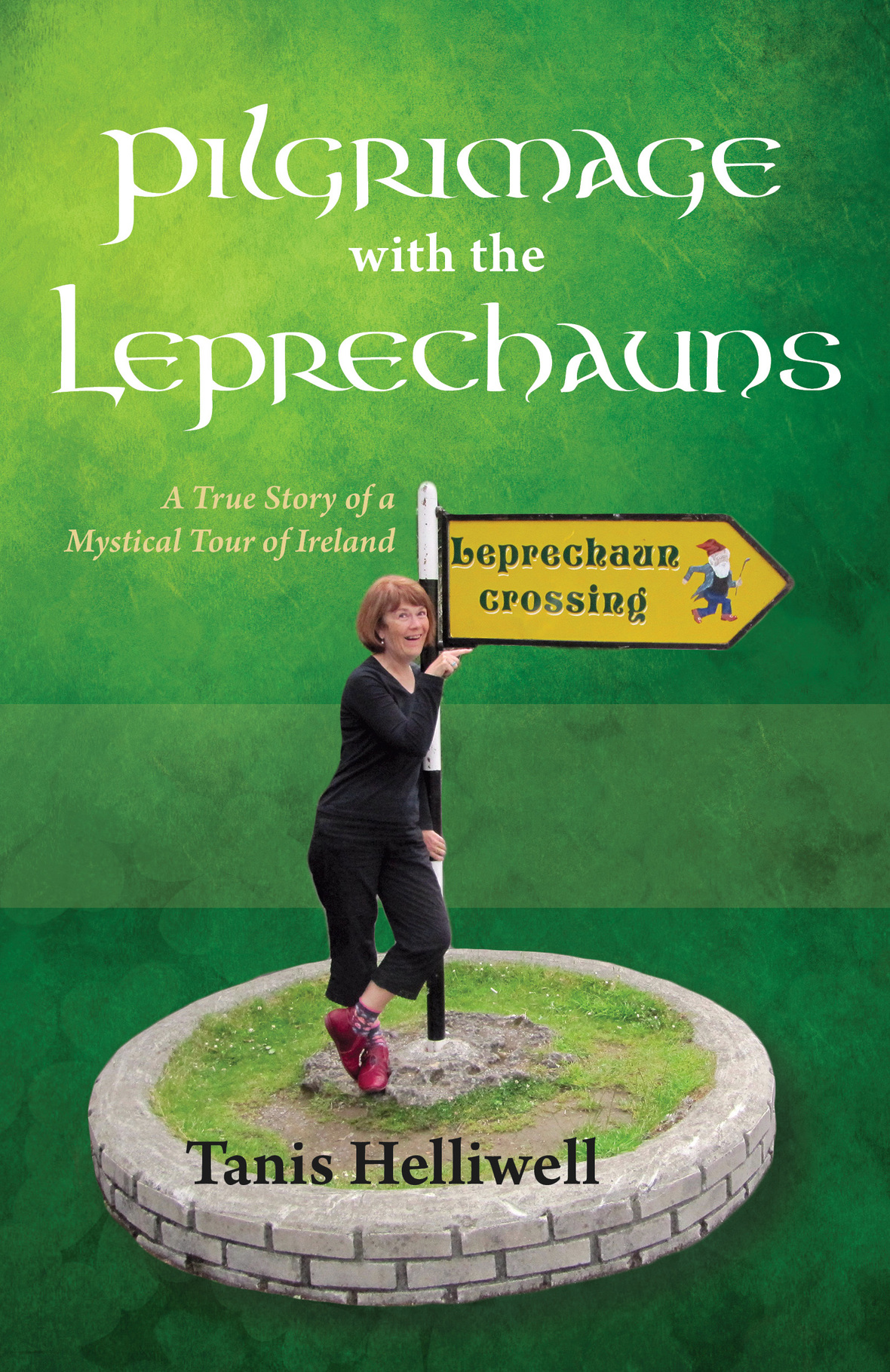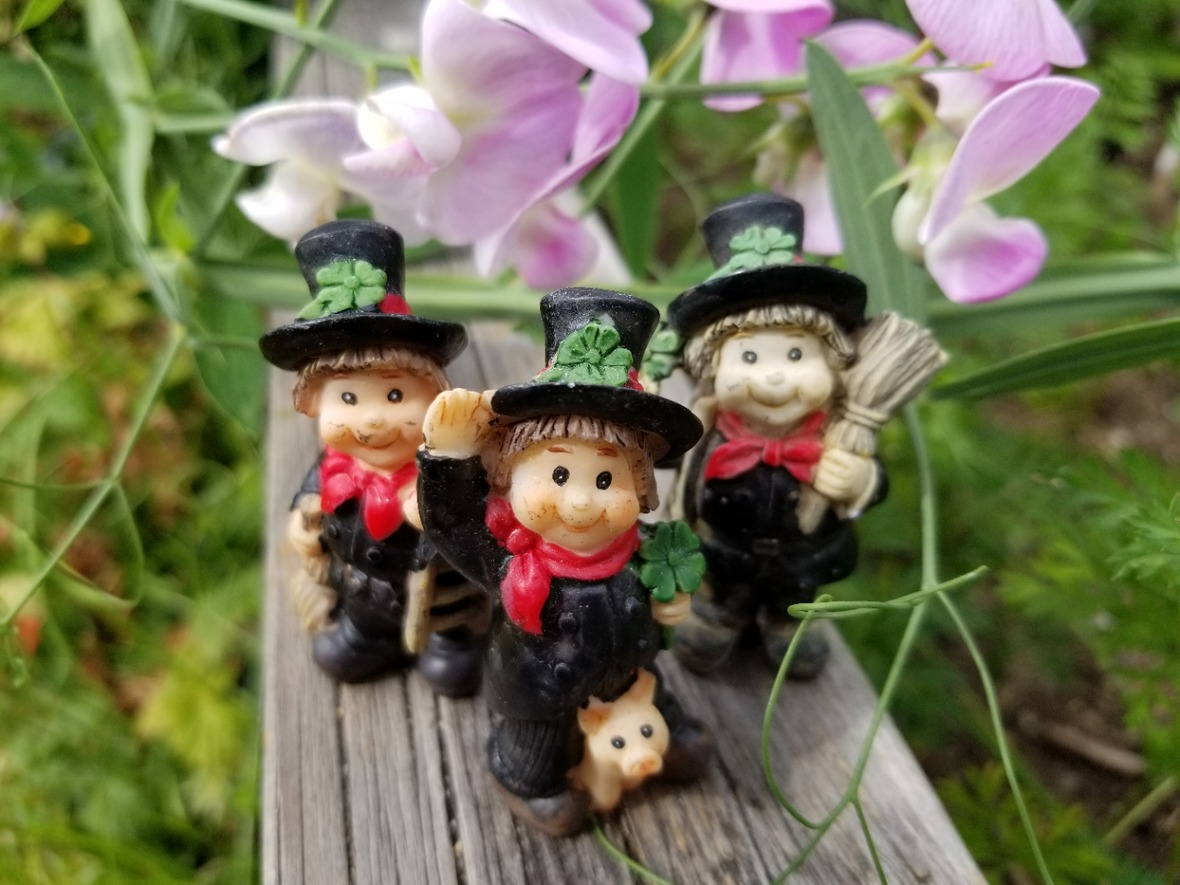# AUGUST 2020

 /* styles */ Dear Friend,
 /* styles */ Another first to announce! My book HYBRIDS: So you think you are human is now available in Italian (print format)! Isn't the cover gorgeous? It is currently available for a special launch price from the publisher, Spazio Interiore. The sale on the new French and Spanish translations of my classic books Summer With the Leprechauns and Pilgrimage With the Leprechauns will continue until the end of August. Be sure to get your copy this month - for yourself or for your friends!
 table div table+table+table+table+table div table{width:100%;padding:0}table div table+table+table+table+table div table img{width:96.23%;padding:0;float:none}table div table+table+table+table+table div table td{width:100%;padding:0 1.88% 18px}/* styles *//* styles */ “A human race made up of a myriad of rainbow hues is being created year by year on this planet, and you and I are a part of this magnificent growth.” --Tanis Helliwell, from Hybrids: So you think you are human
 table div table+table+table+table+table+table+table div table{width:100%;padding:0}table div table+table+table+table+table+table+table div table img{width:96.23%;padding:0;float:none}table div table+table+table+table+table+table+table div table td{width:100%;padding:0 1.88% 18px}/* styles */# Hybrids : Pensi davvero di essere umano?

Miti, religioni e scoperte archeologiche di tutto il mondo evocano la presenza di esseri intelligenti non umani che si sono incrociati con gli umani creando gli ibridi. Hybrids offre informazioni precise e puntuali, corredate da numerose testimonianze, che svelano le caratteristiche delle ventidue tipologie di ibridi umani.

 table div table+table+table+table+table+table+table+table+table+table+table div table{width:100%;padding:0}table div table+table+table+table+table+table+table+table+table+table+table div table img{width:96.23%;padding:0;float:none}table div table+table+table+table+table+table+table+table+table+table+table div table td{width:100%;padding:0 1.88% 18px}/* styles */# HYBRIDS (English) on sale now

The original English edition of Hybrids: So you think you are human is also available for a special price for the month of August. Get your copies here:

US orders use amazon.com | Canadian orders use amazon.ca

 table div table+table+table+table+table+table+table+table+table+table+table+table+table div table{width:100%;padding:0}table div table+table+table+table+table+table+table+table+table+table+table+table+table div table img{width:96.23%;padding:0;float:none}table div table+table+table+table+table+table+table+table+table+table+table+table+table div table td{width:100%;padding:0 1.88% 18px}/* styles */# HYBRIDS: An introduction to the ITALIAN EDITION

View this new YouTube video with Italian sub-titles via the direct link here:

https://youtu.be/46BuBF1akfo

 table div table+table+table+table+table+table+table+table+table+table+table+table+table+table+table div table td,table.module-14{width:100%;padding:0}table div table+table+table+table+table+table+table+table+table+table+table+table+table+table+table div table{width:100%;float:none;margin-left:auto;margin-right:auto;padding:0}table div table+table+table+table+table+table+table+table+table+table+table+table+table+table+table div table a{border:0 none;text-decoration:none}table div table+table+table+table+table+table+table+table+table+table+table+table+table+table+table div table img{width:100%!important;border:0 none;text-decoration:none}/* styles */
 table div table+table+table+table+table+table+table+table+table+table+table+table+table+table+table+table div table{width:100%;padding:0}table div table+table+table+table+table+table+table+table+table+table+table+table+table+table+table+table div table img{width:96.23%;padding:0;float:none}table div table+table+table+table+table+table+table+table+table+table+table+table+table+table+table+table div table td{width:100%;padding:0 1.88% 18px}/* styles */## SALE on leprechaun books continues

Celebrating the new French and Spanish editions, along with the original English editions of Summer With the Leprechauns and Pilgrimage With the Leprechauns, the special launch price will continue for the month of August.

▪ FRENCH BOOKS: Both of my leprechaun books are now available in both print and e-book formats, as Un été avec les Leprechauns (Summer) and Pèlerinage avec les Leprechauns (Pilgrimage).
▪ SPANISH BOOK: My classic book, Summer with the Leprechauns, is now out as an e-book and a thoroughly revised paperback. The Spanish title is Un verano con los Duendes.
▪ ENGLISH BOOK: Pilgrimage with the Leprechauns has a completely new cover more in keeping with the hilarious story - how fun! (The text inside has been revised only very slightly.)
 ▪ FRENCH BOOKS: Both of my leprechaun books are now available in both print and e-book formats, as Un été avec les Leprechauns (Summer) and Pèlerinage avec les Leprechauns (Pilgrimage).
 ▪ SPANISH BOOK: My classic book, Summer with the Leprechauns, is now out as an e-book and a thoroughly revised paperback. The Spanish title is Un verano con los Duendes.
 ▪ ENGLISH BOOK: Pilgrimage with the Leprechauns has a completely new cover more in keeping with the hilarious story - how fun! (The text inside has been revised only very slightly.)

E-BOOKS: \$7.99 (regular \$9.99)

PRINT BOOKS: \$13 (regular \$15)

 table div table+table+table+table+table+table+table+table+table+table+table+table+table+table+table+table+table+table+table div table{width:100%;padding:0}table div table+table+table+table+table+table+table+table+table+table+table+table+table+table+table+table+table+table+table div table img{width:96.23%;padding:0;float:none}table div table+table+table+table+table+table+table+table+table+table+table+table+table+table+table+table+table+table+table div table td{width:100%;padding:0 1.88% 18px}/* styles *//* styles */ View my new video introductions (below) of the translated leprechaun books, with subtitles in French and Spanish! * Remember to click on the "CC" button at bottom right of the video screen in order to activate the captions.
 table div table+table+table+table+table+table+table+table+table+table+table+table+table+table+table+table+table+table+table+table+table+table div table{width:100%;padding:0}table div table+table+table+table+table+table+table+table+table+table+table+table+table+table+table+table+table+table+table+table+table+table div table img{width:96.23%;padding:0;float:none}table div table+table+table+table+table+table+table+table+table+table+table+table+table+table+table+table+table+table+table+table+table+table div table td{width:100%;padding:0 1.88% 18px}/* styles */table div table+table+table+table+table+table+table+table+table+table+table+table+table+table+table+table+table+table+table+table+table+table+table+table div table td,table.module-23{width:100%;padding:0}table div table+table+table+table+table+table+table+table+table+table+table+table+table+table+table+table+table+table+table+table+table+table+table+table div table{width:100%;float:none;margin-left:auto;margin-right:auto;padding:0}table div table+table+table+table+table+table+table+table+table+table+table+table+table+table+table+table+table+table+table+table+table+table+table+table div table a{border:0 none;text-decoration:none}table div table+table+table+table+table+table+table+table+table+table+table+table+table+table+table+table+table+table+table+table+table+table+table+table div table img{width:100%!important;border:0 none;text-decoration:none}/* styles */
 /* styles */ US orders use amazon.com | Canadian orders use amazon.ca
 table div table+table+table+table+table+table+table+table+table+table+table+table+table+table+table+table+table+table+table+table+table+table+table+table+table+table+table div table{width:100%;padding:0}table div table+table+table+table+table+table+table+table+table+table+table+table+table+table+table+table+table+table+table+table+table+table+table+table+table+table+table div table img{width:96.23%;padding:0;float:none}table div table+table+table+table+table+table+table+table+table+table+table+table+table+table+table+table+table+table+table+table+table+table+table+table+table+table+table div table td{width:100%;padding:0 1.88% 18px}/* styles */table div table+table+table+table+table+table+table+table+table+table+table+table+table+table+table+table+table+table+table+table+table+table+table+table+table+table+table+table+table div table td,table.module-28{width:100%;padding:0}table div table+table+table+table+table+table+table+table+table+table+table+table+table+table+table+table+table+table+table+table+table+table+table+table+table+table+table+table+table div table{width:100%;float:none;margin-left:auto;margin-right:auto;padding:0}table div table+table+table+table+table+table+table+table+table+table+table+table+table+table+table+table+table+table+table+table+table+table+table+table+table+table+table+table+table div table a{border:0 none;text-decoration:none}table div table+table+table+table+table+table+table+table+table+table+table+table+table+table+table+table+table+table+table+table+table+table+table+table+table+table+table+table+table div table img{width:100%!important;border:0 none;text-decoration:none}/* styles */
 /* styles */ US orders use amazon.com | Canadian orders use amazon.ca
 table div table+table+table+table+table+table+table+table+table+table+table+table+table+table+table+table+table+table+table+table+table+table+table+table+table+table+table+table+table+table+table+table div table{width:100%;padding:0}table div table+table+table+table+table+table+table+table+table+table+table+table+table+table+table+table+table+table+table+table+table+table+table+table+table+table+table+table+table+table+table+table div table img{width:96.23%;padding:0;float:none}table div table+table+table+table+table+table+table+table+table+table+table+table+table+table+table+table+table+table+table+table+table+table+table+table+table+table+table+table+table+table+table+table div table td{width:100%;padding:0 1.88% 18px}/* styles *//* styles */AN IRISH JEWEL, far more than a fairy tale. A good humored, true account of Tanis’ summer in a cottage in Ireland where the resident leprechaun taught her about the evolution of elementals (fairies, elves, leprechauns). Learn about the importance of humans and elementals working together for the betterment of both of their races and the Earth.(NEW COVER ONLY) ... FULL OF INFORMATION about nature spirits or elementals, as they like to call themselves. The trolls, elves, goblins, and brownies are as real for the reader as the various humans whom they partner. For anyone planning a trip to the sacred sites of Ireland, or to Ireland itself for that matter, this book abounds in nuggets as precious as the pot of gold that the Leprechaun holds at the end of the rainbow.

▪ Summer with the Leprechauns (there have been no changes to this book in English!)
▪ Pilgrimage with the Leprechauns (new cover only)
 ▪ Summer with the Leprechauns (there have been no changes to this book in English!)
 ▪ Pilgrimage with the Leprechauns (new cover only)
 table div table+table+table+table+table+table+table+table+table+table+table+table+table+table+table+table+table+table+table+table+table+table+table+table+table+table+table+table+table+table+table+table+table+table+table+table div table{width:100%;padding:0}table div table+table+table+table+table+table+table+table+table+table+table+table+table+table+table+table+table+table+table+table+table+table+table+table+table+table+table+table+table+table+table+table+table+table+table+table div table img{width:96.23%;padding:0;float:none}table div table+table+table+table+table+table+table+table+table+table+table+table+table+table+table+table+table+table+table+table+table+table+table+table+table+table+table+table+table+table+table+table+table+table+table+table div table td{width:100%;padding:0 1.88% 18px}/* styles */table div table+table+table+table+table+table+table+table+table+table+table+table+table+table+table+table+table+table+table+table+table+table+table+table+table+table+table+table+table+table+table+table+table+table+table+table+table+table+table+table div table{width:100%;padding:0}table div table+table+table+table+table+table+table+table+table+table+table+table+table+table+table+table+table+table+table+table+table+table+table+table+table+table+table+table+table+table+table+table+table+table+table+table+table+table+table+table div table img{width:96.23%;padding:0;float:none}table div table+table+table+table+table+table+table+table+table+table+table+table+table+table+table+table+table+table+table+table+table+table+table+table+table+table+table+table+table+table+table+table+table+table+table+table+table+table+table+table div table td{width:100%;padding:0 1.88% 18px}/* styles */